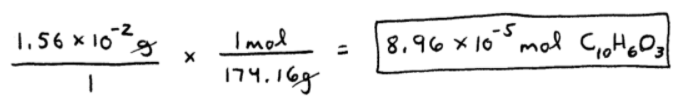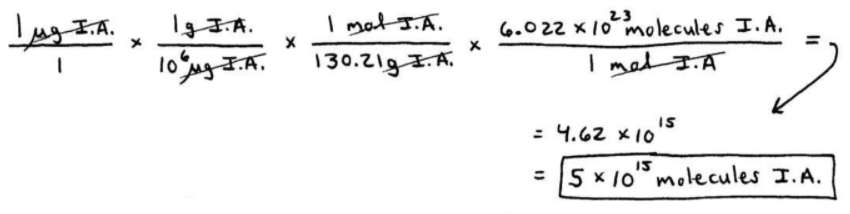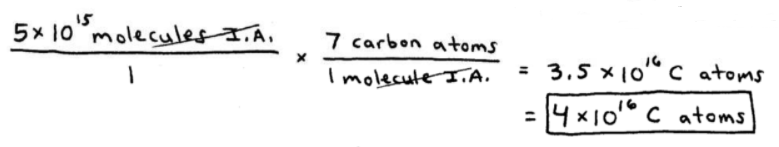# S3E2 - How to Find Molar Mass, Grams-to-Moles, and Grams-to-Molecules

## How to Calculate Molar Mass

Molar Mass  =  the mass of 1 mole of a compound.

➞ often referred to as "molecular weight" or "formula mass."

➞ just like an element's atomic mass (i.e. 12.01 g/mol for C), compounds and molecules have a molar mass.

Let's do a molar mass calculation example...

==========

## How Do You Find Molar Mass?

We'll see how to calculate the molar mass in the following example...

----------

ex:  A chemical dye has the formula C10H6O3

Calculate the molar mass of this compound.
_________

➞  we'll need to use the Periodic Table:

10 C  =  10 (12.01)  =  120.10
6 H  =  6 (1.01)  =  6.06
3 O  =  3 (16.00)  =  48.00

So, the total amounts to the molar mass of C10H6O3  =

=  174.16 g/mol

==========

## How to Find Moles from Grams

What if we wanted to learn how to find moles from grams?

Let's take a look below...

----------

ex:  A chemical dye has the formula C10H6O3

How many moles of C10H6O3 are contained in 1.56 x 10-2 g of the dye?
_________

➞  "start with what you're given, and put it over 1" (remember?)...(1.56 x 10-2 g / 1) . (1 mole / 174.16 g)  =

=  8.96 x 10-5 mol C10H6O3

==========

## Grams to Molecules Calculations

Though not as common as grams-to-moles and moles-to-grams conversions, knowing how to do grams-to-molecules calculations is also extremely important.

Check out the grams-to-molecules example below.

----------

ex Isopentyl acetate, C7H14O, gives bananas their smell.

If a bee sting releases 1 µg of the compound, how many molecules is this?

How many carbon atoms is this?
_________

➞  Before we start, we'll need the molar mass:

7 C  =  7 (12.01)  =  84.07
14 H  =  14 (1.01)  =  14.14
2 O  =  2 (16.00)  =  32.00

So, the total amounts to the molar mass of C7H14O2 =

130.21 g/mol

Next, we'll convert 1 µg to molecules in 3 steps.Okay, lastly we just have to count the number of carbon atoms contained in a single molecule of isopentyl acetate (I.A.):==========

## How to Calculate Mass Percent

Mass Percent  =  an element's percentage of the total mass of a compound containing the element.

➞ also called percent composition or mass percent composition.

➞ let's use Isopentyl Acetate, C7H14O2 , from the previous example.

See the image below.

Here's how we calculate mass percent:As you can see, isopentyl acetate is:

64.6% carbon by mass,
24.5% oxygen by mass, and
10.9% hydrogen by mass.
_________
100% total

==========

Next time, in our 3rd set of video notes from SECTION 3 - Chemical Quantities and Stoichiometry,

We'll talk about How to Determine Empirical and Molecular Formula...